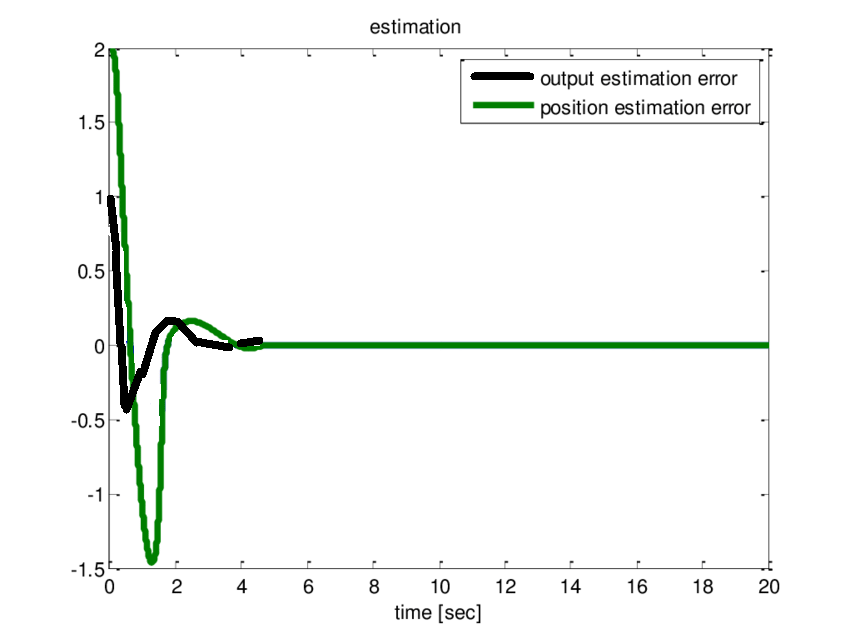# Software Engineering | Curve Fitting models

The curve fitting group models use statistical regression analysis to study the relationship between software complexity and the number of faults in a program, the number of changes, or failure rate. This group of models finds a relationship between input and output variables by using the methods linear regression, nonlinear regression, or time series analysis. The dependent variables, for example, are the number of errors in a program. The independent variables are the number of modules changed in the maintenance phase, the time between failures, programmers’ skill, program size, etc. Models included in this group are: Estimation of errors, Estimation of complexity, Estimation of failure rate. These are explained as following below.

Below is the graph b/w actual vs estimation.1. Estimation of Errors Model:
The number of errors in a program can be estimated by using a linear or nonlinear regression model. A simple nonlinear regression model to estimate the total number of initial errors in the program, N, can be presented as follows:where Xi is the ith error factor; ai, bi, ci are the coefficients of the model, andis an error term. Typical error factors are software complexity metrics and environmental factors. Most curve fitting models involve only one error factor.

2. Estimation of Complexity Model:
This model is used to estimate the software complexity, CR, using the time series approach. The software complexity model is summarized as follows:where
R = release sequence number
ER = environmental factor(s) at release R
MR = number of modules at release R
IR = inter-release interval R
D = number of days when first error occurs= error

This perticular model is used when the software is evaluated due to time by time means when more versions of the model are released.

3. Estimation of Failure Rate Model:
THis model is used to estimate the failure rate of software. Given failure times t1, t2, .., tn, a rough estimate of the failure rate at the ith failure interval isAssuming that the failure rate is monotonically non-increasing, an estimate of this function, i = 1, 2, …, n can be obtained by using the least squared method.

My Personal Notes arrow_drop_upCheck out this Author's contributed articles.

If you like GeeksforGeeks and would like to contribute, you can also write an article using contribute.geeksforgeeks.org or mail your article to contribute@geeksforgeeks.org. See your article appearing on the GeeksforGeeks main page and help other Geeks.

Please Improve this article if you find anything incorrect by clicking on the "Improve Article" button below.

Article Tags :

Be the First to upvote.

Please write to us at contribute@geeksforgeeks.org to report any issue with the above content.